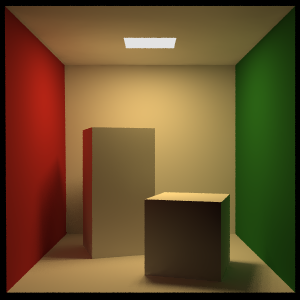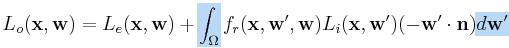# Lighting: The Rendering Equation

Back in 2002, I started my first job in the games industry at Climax Studios in England. I have to admit, I didn’t know very much about game development at the time. Don’t get me wrong, I’d been writing little 2D games, and messing around with rubbish particle systems at home, but it was nothing like what I was about to get involved with. Despite my inexperience, somehow I did enough to pass the interview, and I was offered a job as a junior programmer.

As seemed to be typical for the time, my introduction to the industry was pretty much a trial by fire. I quickly found out that I couldn’t hope to truly understand every single new thing I encountered, so I learned to just accept some things as the truth. For example, I was told that the dot product of two normalized vectors yields the cosine of the angle between them. I just accepted this, and only took the time to find out why later on.One of the things I accepted at the beginning was the maths used to perform the lighting of our models during rendering. While I could understand how the equations appeared to yield decent looking results, I never understood where they came from, and why they worked.

After a while I began to wonder about this… Where did the equations for diffuse and specular reflections come from? What are the units of brightness we use for lights? What are the units for the pixels that get rendered?

It took lot of reading, re-reading, and re-re-reading, for me to really understand some of these things, so now that I have a blog, I thought I would share what I learned just in case anyone else is wondering about these things too.

## The Rendering Equation

When light hits a point on a surface, some of it might get absorbed, reflected or possibly even refracted. Also, there may be additional light being emitted from that point by a power source, or perhaps scattered in from another point on the surface. Things can get complicated pretty quickly!

Luckily for us, some smart guys came up with something called the rendering equation to deal with these factors. The rendering equation can produce incredibly realistic-looking images, but in its original form, it can also be very costly to evaluate.I’ve included a slightly simplified version of the rendering equation below. If you compare this to the version currently on Wikipedia, you’ll see that I’ve removed a couple of parameters, t and lamba.

Typically if you have something like the power output of a light varying over time, it’s a better to idea to evaluate it before trying to solve the rendering equation. By doing this, you can assume time is constant, and so you can ignore it.

The lambda symbol in the original equation represents a dependency on the wavelength of the light. Without this, everything would be greyscale, so it’s an important property. Rather than dealing with wavelength explicitly, we can just treat the red, green and blue color channels independently and solve the rendering equation once for each channel. Note that in practice we end up using per-component vector mathematics to solve the equation for all three channels at the same time.At first, this may seem a little bit intimidating, but actually it’s fairly simple. I’m going to break down each part and explain what it means.

Outgoing LightThis says that the rendering equation is a function which gives you the outgoing light in a particular direction w from a point x on a surface.

Emitted LightThis is any light that is being emitted from the point. Most surfaces don’t emit light, so normally you don’t see any contribution here.

IntegralThis says that the enclosed functions need to be integrated over all directions w’ in the hemisphere above x. The orientation of the hemisphere is determined by the normal, n.

BRDFThis is the bidirectional reflectance distribution function (BRDF). It’s a fancy name for the ratio of the amount of light reflected in a particular direction w, to the amount received from another direction w’. The BRDF warrants its own discussion, but for now it can just be thought of as the reflection amount.

Incoming LightThis is the incoming light at the point x from the direction w’. Note that the incoming light doesn’t have to come from a light source (direct light). It may have been reflected or refracted from another point in the scene (indirect light).

Normal AttenuationThis attenuates the incoming light at x based on the cosine of the angle between the normal n and the incoming light direction w’.

## Making it Real-Time

We use the rendering equation to perform lighting calculations in games, albeit in a simpler form. The most obvious problem for evaluating the rendering equation in a pixel shader is the integral, so we need to find a way to approximate it. One thing we can do is to split the way we deal with direct light and indirect light.

Since the indirect light is the harder of the two to deal with, we can approximate it. There are various different ways you might want to approximate the indirect light, from a simple ambient color, to more complex forms like spherical harmonics. Typically you would modulate your indirect light approximation with the diffuse part of your BRDF, since you don’t have a directional component to use in direction-dependent BRDFs.

By approximating the indirect light, we only have to worry the direct light in the rendering equation, so we can replace the integral with a simple sum over k light sources. Also, we typically model the change in the BRDF over space by using textures mapped over the surface, so x is no longer needed for that function.

I’ve changed up the notation here to match more closely what you see in games literature. The vector v is the normalized direction from the point x to the camera. The vector li is the normalized vector from light source i to the point x.It’s not explicitly written here, but the dot product now needs to be clamped to zero to avoid negative light values.

This may not look much like the ‘diffuse plus specular’ equations you commonly see in games, but it’s actually pretty close. In fact, imagine removing the emitted light, and swapping the BRDF function with a constant color value, and you’ll see that we have the equation for calculating diffuse lighting.The multiply symbol inside the circle just represents the component-wise multiplication of the red, green and blue channels of the color with the corresponding channels of the incoming light.

## Units…

You may notice that I’ve been using fuzzy terms like ‘incoming light’ as if it’s something we all know how to measure. A good question at this point might be “what are the units being used for light?”.Well, there are well defined units at play here, but I’m going to say right now that the units don’t really matter. I don’t know of any game engines where they attribute real-world units to lighting and material values, since those kinds of things tend to be driven more by the appearance than the physical correctness. The relative intensities of, say, a candle and a 100W light bulb are probably more important than the absolute values.

Having said all that, here’s the information anyway: The light is measured in units of radiance (Wsr-1m-2). Looking back at the integral in the rendering equation, you can see that the radiance is multiplied by the differential solid angle. This converts the radiance into irradiance (Wm-2).

Remember that the BRDF is the ratio of light reflected to light received? Well that’s a ratio of radiance to irradiance, so the BRDF has units of sr-1.

One thing to be careful of is that the incoming light in the simplified version of the rendering equation (using the sum over the lights) is measured in units of irradiance (Wm-2). We have to use irradiance directly here since the typical lights we use (point, spot, directional) don’t have any area.

## That’s It For Now

I hope what I’ve explained so far was fairly clear, but I know I’ve left some pretty big holes here. I’m going to be looking at some of the following at a later date:

• What is the BRDF?
• What is energy conservation, and does it matter for games?

## 2 thoughts on “Lighting: The Rendering Equation”

1.howler

nicely explained, i’m a 3rd year games development student and this has particular relevance to me. thanks again…..HowleR

2.Tom

Hi,
thanks a lot for this post, an extremely useful introduction to the rendering equation (the math can be intimidating without anyone to walk you through it).

I have a question though – how does the rendering equation account for refraction, exactly? I see it integrates the incoming irradiance over the hemisphere centered on the normal, but a ray being refracted could be coming from outside this hemisphere (suppose you were considering a glass surface).

Do we need to integrate the whole sphere in that case? Or perhaps just the hemisphere plus the refraction angle as per Snell’s Law? And how would this affect energy conservation? I am confused.

Thanks.